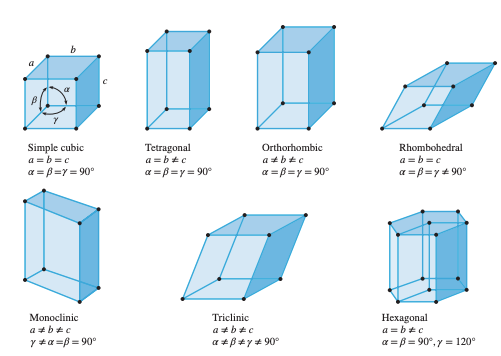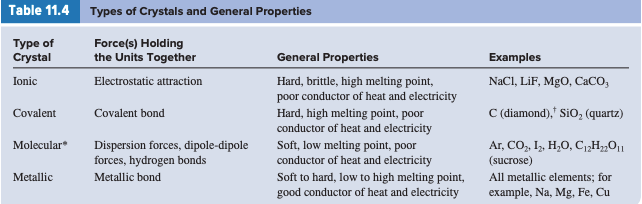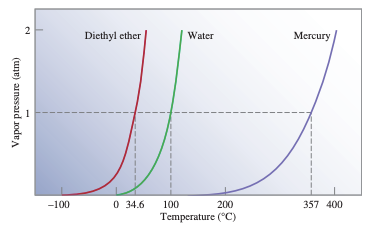# 11.1 - The Kinetic Molecular Theory of Liquids and Solids

• Phases are the multiple states of a chemical that exist in a system, according to chemists.

• A phase is a homogeneous part of a system that is in contact with other sections but separated from them by a well-defined border.

# 11.2 - Intermolecular Forces

• The non-ideal behavior of gases is caused by intermolecular forces.

• Intermolecular forces are forces that attract molecules together.

• This transition from the gaseous to the liquid phase is known as condensation

• Intramolecular forces, in contrast to intermolecular forces, hold atoms in a molecule together.

• What chemists call van der Waals forces are dipole-dipole, dipole-induced dipole, and dispersion forces, named after the Dutch physicist Johannes van der Waals.

• Dipole-dipole forces are attractive forces that exist between polar molecules, or molecules with dipole moments.

• Ion-dipole forces, which attract an ion and a polar molecule to each other, are likewise explained by Coulomb's law.

• Because the separation of positive and negative charges in the atom is caused by the vicinity of an ion or a polar molecule, the dipole in the atom is called an induced dipole.

• Ion-induced dipole interaction is the attractive interaction between an ion and the induced dipole dipole-induced dipole interaction is the attractive interaction between a polar molecule and the induced dipole.

• The crucial point is that dispersion forces, or attractive forces, are produced as a result of transient dipoles formed in atoms or molecules in this type of contact.

# 11.3 - Properties of Liquids

• Surface tension is a measurement of the elastic force on a liquid's surface.

• The amount of energy required to stretch or raise the surface area of a liquid by a unit area is known as surface tension.

• Capillary action is caused by two types of forces.

• The first is cohesion, which is the intermolecular attraction between molecules that are similar.

• Adhesion is a force that attracts molecules that are unlike each other, such as those in water and those in the sides of a glass tube.

• The idiom "slow as molasses in January" is based on a physical feature of liquids known as viscosity.

• The viscosity of a fluid is a measurement of its resistance to flow.

# 11.4 - Crystal Structure

• Crystalline and amorphous solids are the two types of solids.

• Ice is a crystalline solid with a rigid and long-range organization; its atoms, molecules, and ions are arranged in specific locations.

• A crystalline solid basic repeating structural unit is the unit cell.

• The number of atoms around an atom in a crystal lattice is known as the coordination number.

• A simple cubic cell is the simplest, repeating unit in the array of spheres.

• The body-centered cubic cell and the face-centered cubic cell are the other types of cubic cells.# 11.5 - X-Ray Diffraction by Crystals

• X-ray diffraction investigations have taught us almost what we know about crystal structure.

• The scattering of X rays by the units of a crystalline material is known as X-ray diffraction.

# 11.6 - Types of Crystals

• Ionic crystals have two distinguishing features

• (1) they are made up of charged species

• (2) the sizes of anions and cations are often significantly varied.# 11.7 - Amorphous Solids

• Glass and other amorphous materials lack a regular three-dimensional arrangement of atoms.

• Amorphous refers to the resulting solid.

• Glass is one of the most valuable and adaptable materials in human history.

• It's also one among the oldest, with glass artifacts dating back to 1000 B.C.

• An optically transparent fusion product of inorganic materials that has cooled to a rigid state without crystallizing is frequently referred to as glass.# 11.8 - Phase Changes

• When energy is added or removed from a substance, phase shifts, or transformations from one phase to another, occur.

• A phase transition occurs when the molecules in a liquid have enough energy to escape from the surface.

• Evaporation, often known as vaporization, is the transformation of a liquid into a gas.

• Condensation, or the transition from gas to liquid, occurs when a molecule collides with the liquid surface and is confined by intermolecular forces in the liquid.

• When the rates of condensation and evaporation are equal, a condition of dynamic equilibrium is established

• The rate of a forward process is exactly balanced by the rate of the reverse process.

• The equilibrium vapor pressure is the vapor pressure measured when condensation and evaporation are in a dynamic equilibrium.

• The molar heat of vaporization (Hvar), defined as the energy (typically in kilojoules) required to evaporate 1 mole of a liquid, is a measure of the strength of intermolecular forces in a liquid.• The temperature at which a liquid's vapor pressure equals the external pressure is known as the boiling point.

• When the external pressure is 1 atm, a liquid's normal boiling point is the temperature at which it boils.

• No matter how high the applied pressure, every substance has a critical temperature (Tc) above which its gas phase will not liquefy.

• The critical pressure (Pc) is the lowest pressure required to cause liquefaction at the critical temperature.

• Freezing is the process of turning a liquid into a solid and melting, or fusing is the process of turning a solid into a liquid.

• The temperature at which solid and liquid phases coexist in equilibrium is known as the melting point of a solid or the freezing point of a liquid.

• Supercooling is a phenomenon in which a liquid can be chilled to below its freezing point for a short time.

• Sublimation is the transition of molecules from the solid-state to the vapor state.

• A deposition is the inverse process, in which molecules go directly from vapor to solid.

• The energy necessary to sublime 1 mole of a solid is known as molar heat of sublimation (Hsub).

# 11.9 - Phase Diagrams

• A phase diagram is a single graph that depicts the general relationships between the solid, liquid, and vapor phases.

• The conditions under which a substance exists as a solid, liquid, or gas are summarized in a phase diagram.

• The triple point, which is the only situation in which all three phases can be in equilibrium with one another, is where all three curves meet.